## Block Growth

Letbe a sequence over a finite Alphabet(all the entries are elements of). Define the block growth functionof a sequence to be the number of Admissible words of length. For example, in the sequence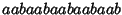..., the following words are Admissible

 Length Admissible Words 12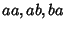34so,,,, and so on. Notice that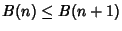, so the block growth function is always nondecreasing. This is because any Admissible word of lengthcan be extended rightwards to produce an Admissible word of length. Moreover, suppose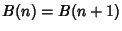for some. Then each admissible word of lengthextends to a unique Admissible word of length.

For a Sequence in which each substring of lengthuniquely determines the next symbol in the Sequence, there are only finitely many strings of length, so the process must eventually cycle and the Sequence must be eventually periodic. This gives us the following theorems:

1. If the Sequence is eventually periodic, with least period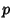, thenis strictly increasing until it reaches, andis constant thereafter.

2. If the Sequence is not eventually periodic, thenis strictly increasing and sofor all. If a Sequence has the property that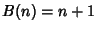for all, then it is said to have minimal block growth, and the Sequence is called a Sturmian Sequence.

The block growth is also called the Growth Function or the Complexity of a Sequence.